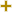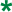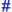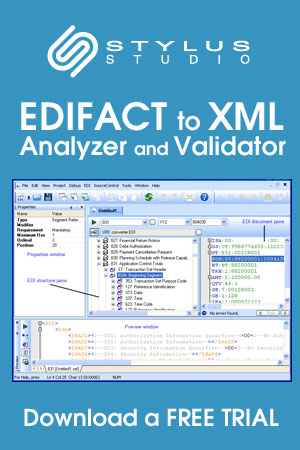Return to Stylus Studio EDIFACT D04A Code List page.

Price multiplier type code qualifierUN/CEFACT Revision 2004A Code ListRepr:an..3
Desc:Code qualifying the type of price multiplier.
This table also occurs in the following versions of this standard:
D00A, D00B, D01A, D01B, D01C, D02A, D02B, D03A, D03B, D04A, D04B, D93A, D94A, D94B, D95A, D95B, D96A, D96B, D97A, D97B, D98A, D98B, D99A, D99B

 A Price adjustment coefficient Coefficient to be used in the price adjustment formula to calculate the revaluated price. B Escalation coefficient Coefficient to be used in the escalation formula to calculate the actual price. C Timesing factor Factor to be used in calculating the number of times a particular piece of work is repeated. CSD Cost markup multiplier - original cost Code specifying the cost mark-up multiplier at original cost. CSR Cost markup multiplier - retail cost Code specifying the cost mark-up multiplier at retail cost. DIS Discount multiplier Code specifying the discount multiplier. SEL Selling multiplier Code specifying the selling multiplier.

Change indicators
plus signAn addition.
asteriskAddition/substraction/change to a code entry for a particular data element.
hash or pound signChanges to names.
vertical barChanges to text for descriptions, notes and functions.
minus signA deletion.
letter XMarked for deletion.

Usage indicatorsUsed in batch messages only.Common usage in both batch and interactive messages.Used in interactive messages only.

Return to Stylus Studio EDIFACT D04A Code List page.